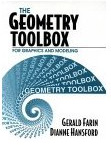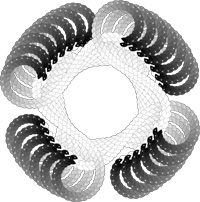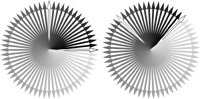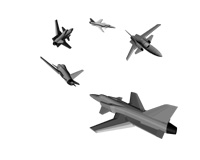No copies of The Geometry Toolbox are left! It has been replaced by Practical Linear Algebra - A Geometry Toolbox We have added material which makes this a suitable text for a broader offering of Linear Algebra courses geared to engineers, scientists, and practitioners. As with the original text, we take geometric and intuitive approach to Linear Algebra.The Geometry Toolbox
for Graphics and Modeling

by Gerald Farin & Dianne Hansford

288 pages, ISBN: 1-56881-074-1

The Geometry Toolbox introduces you to the basic concepts of linear algebra and geometry. You will find it useful if you are in computer graphics or geometric modeling and feel that your geometry skills are a bit rusty. Mainly, however, it is a geometric introduction to linear algebra. The book has over 250 figures, over 100 exercises with many solved at the end of the book, and over 100 numerical examples.

The figures are not included as window dressing, in fact they play an important role in bringing the reader to a robust understanding of the mathematics. However they are not only instructional, they are also fun!

For example, on the right is a crazy Pacman path -- created with linear transformations and a bit of coloration thanks to Postscript

Below, left is an instructional tool used to demonstrate a rotation by 45 degree, and below right is simply a fun example of what we can do with 3D transformations.German translation:

Linear Algebra: A geometric introduction

Published in 2003 by Springer Verlag, Heidelberg